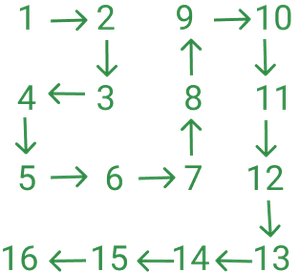Open in App
Not now

# Find the element at specified index in a Spiral Matrix

• Last Updated : 26 Apr, 2021

Given two integers i and j, the task is to print the i * j thmatrix elements that can be obtained by filling the matrix in the following spiral manner:Spiral Grid Representation fig 1

Examples:

Input: i = 3, j = 4
Output: 12
Explanation:
i = 3, j = 4 and grid = 12

Input: i = 5, j = 5
Output: 21
Explanation:
i = 5, j = 5 grid = 21

Approach: In the problem, it can be observed that when i is even the first number in the grid is i2 (2 = 4 in 2nd row), and when i is the odd first number in the grid is (i-1)2 + 1 ((3-1)2 + 1 = 5 in 3rd row). Similarly, when j is odd the first number in the grid is j2 (32 = 9 in 3rd column) and when j is even first number in the grid is (j-1)2 + 1 ((4-1)2 + 1 = 10 in 4th column). So every row starts with either the i2 or (i-1)2 + 1 and every column starts with either j2  or (j-1)2 + 1

The problem can be divided into the following cases :

• Case 1 : i = j
Observe that diagonal elements of the grid can be represented by the formula i2 – (i-1) or j2 – (j – 1).
• Case 2: i > j
• Case 1: i is even
In this case, the first number of row i will be i2. Now by subtracting (j – 1) from the first number of the row calculate the value present at the given index. So the formula will be i – (j-1).
• Case 2: i is odd
In this case, the first number of row i will be (i – 1)2 + 1. Now by adding (j – 1) to the first number of the row calculate the value present at the given index. So the formula will be (i – 1)2 + 1 + (j – 1).
• Case 3: i < j
• Case 1: j is even
In this case, the first number of column j will be (j – 1)2 + 1. Now by adding (i – 1) to the first number of the column calculate the value present at the given index. So the formula will be (j – 1)2 + 1 + (i – 1).
• Case 2: j is odd
In this case, the first number of column j will be j2. Now by subtracting (i – 1) from the first number of the column calculate the value present at the given index. So the formula will be j2 + 1 – (i – 1).

Follow the steps below to solve the problem:

1. Check if i is equal to j and print i * i – (i – 1).
2. If i is greater than j:
1. If i is even print i * i – (j – 1).
2. Otherwise, print (i – 1)* (i – 1) + 1 + (j – 1).
3. If j is greater than i:
1. If j is even print (j – 1) * (j – 1) + 1 + (i – 1).
2. Otherwise, print j * j – (i – 1).

Below is the implementation of the above approach:

## C++

 `// C++ program for the above approach``#include ``using` `namespace` `std;` `// Function to the find``// element at (i, j) index``int` `findInGrid(``int` `i, ``int` `j)``{``    ``if` `(i == j)``        ``return` `(i * i - (i - 1));` `    ``else` `if` `(i > j) {``        ``if` `(i % 2 == 0)``            ``return` `i * i - (j - 1);``        ``else``            ``return` `(i - 1) * (i - 1) + 1 + (j - 1);``    ``}` `    ``else` `{``        ``if` `(j % 2 == 0)``            ``return` `(j - 1) * (j - 1) + 1 + (i - 1);``        ``else``            ``return` `j * j - (i - 1);``    ``}``}` `// Driver Code``int` `main()``{` `    ``int` `i = 3, j = 4;` `    ``// Function Call``    ``cout << findInGrid(i, j);` `    ``return` `0;``}`

## Java

 `// Java program for the above approach``import` `java.io.*;` `class` `GFG{` `// Function to the find``// element at (i, j) index``static` `int` `findInGrid(``int` `i, ``int` `j)``{``    ``if` `(i == j)``        ``return` `(i * i - (i - ``1``));` `    ``else` `if` `(i > j)``    ``{``        ``if` `(i % ``2` `== ``0``)``            ``return` `i * i - (j - ``1``);``        ``else``            ``return` `(i - ``1``) * (i - ``1``) +``                         ``1` `+ (j - ``1``);``    ``}` `    ``else``    ``{``        ``if` `(j % ``2` `== ``0``)``            ``return` `(j - ``1``) * (j - ``1``) +``                         ``1` `+ (i - ``1``);``        ``else``            ``return` `j * j - (i - ``1``);``    ``}``}` `// Driver Code``public` `static` `void` `main(String[] args)``{``    ``int` `i = ``3``, j = ``4``;` `    ``// Function Call``    ``System.out.println(findInGrid(i, j));``}``}` `// This code is contributed by Dharanendra L V`

## Python3

 `# Python3 program for the above approach` `# Function to the find``# element at(i, j) index``def` `findInGrid(i, j):``    ` `    ``if` `(i ``=``=` `j):``        ``return` `(i ``*` `i ``-` `(i ``-` `1``))` `    ``elif` `(i > j):``        ``if` `(i ``%` `2` `=``=` `0``):``            ``return` `i ``*` `i ``-` `(j ``-` `1``)``        ``else` `:``            ``return` `((i ``-` `1``) ``*` `(i ``-` `1``) ``+``                          ``1` `+` `(j ``-` `1``))` `    ``else``:``        ``if` `(j ``%` `2` `=``=` `0``):``            ``return` `((j ``-` `1``) ``*` `(j ``-` `1``) ``+``                          ``1` `+` `(i ``-` `1``))``        ``else``:``            ``return` `j ``*` `j ``-` `(i ``-` `1``)` `# Driver Code``i ``=` `3``j ``=` `4` `# Function Call``print``(findInGrid(i, j))` `# This code is contributed by Dharanendra L V`

## C#

 `// C# program for the above approach``using` `System;` `class` `GFG{` `// Function to the find``// element at (i, j) index``static` `int` `findInGrid(``int` `i, ``int` `j)``{``    ``if` `(i == j)``        ``return` `(i * i - (i - 1));` `    ``else` `if` `(i > j)``    ``{``        ``if` `(i % 2 == 0)``            ``return` `i * i - (j - 1);``        ``else``            ``return` `(i - 1) * (i - 1) +``                         ``1 + (j - 1);``    ``}` `    ``else``    ``{``        ``if` `(j % 2 == 0)``            ``return` `(j - 1) * (j - 1) +``                         ``1 + (i - 1);``        ``else``            ``return` `j * j - (i - 1);``    ``}``}` `// Driver Code``static` `public` `void` `Main()``{``    ``int` `i = 3, j = 4;``    ` `    ``// Function Call``    ``Console.WriteLine(findInGrid(i, j));``}``}` `// This code is contributed by Dharanendra L V`

## Javascript

 ``

Output

`12`

Time complexity: O(1)
Auxiliary Space: O(1)

My Personal Notes arrow_drop_up